Jun Wang Chair Professor of Computational Intelligence  Room B6413, Academic Building 1 Department of Computer Science College of Science and Engineering City University of Hong Kong Kowloon Tong, Kowloon, Hong KongTel:  (852) 34429701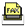Fax: (852) 34420503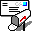jwang.cs@cityu.edu.hk##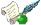EXPERIENCE##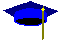EDUCATION##DISTINCTIONS##RESEARCH INTERESTS

• Neural computation
• Computational intelligence
• Optimization methods
• Intelligent control
• Intelligent robotics
• Intelligent manufacturing
• Intelligent data processing##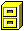MAJOR GRANTS

• General Research Fund: Intelligent motion control and planning of autonomous underwater vehicles, Hong Kong Research Grants Council, 2014-2017.
• General Research Fund: Neurodynamic approaches to constrained optimization with generalized-convex and multiple-objective functions, Hong Kong Research Grants Council, 2013-2016.
• General Research Fund: Analysis and design of memristive neurodynamic systems, Hong Kong Research Grants Council, 2011-2013.
• General Research Fund: Analysis and design of model predictive control based on recurrent neural networks, Hong Kong Research Grants Council, 2009-2012.
• General Research Fund: Analysis and design of associative memories based on recurrent neural networks, Hong Kong Research Grants Council, 2008-2010.
• Earmarked Grant: Sequential blind source extraction from instantaneous and convolutive mixtures for ill-conditioned mixing channels, Hong Kong Research Grants Council, 2004-2006.
• Earmarked Grant: Analysis and design of recurrent neural networks for dynamic optimization subject to nonlinear constraints and their engineering applications, Hong Kong Research Grants Council, 2003-2006.
• Earmarked Grant: Recurrent neural networks for real-time grasping force optimization of dexterous manipulations using multi-fingered robotic hands, Hong Kong Research Grants Council, 2000-2003.
• Earmarked Grant: Multilayer recurrent neural networks for real-time optimization and their applications to optimal control of kinematically redundant manipulators,1998-2001.
• Earmarked Grant: Multilayer recurrent neural networks for synthesizing and optimizing robust linear and nonlinear control systems, Hong Kong Research Council, 1997-2000.
• Earmarked Grant: Recurrent neural network for solving combinatorial optimization with time-varying parameters and their applications for en-route vehicle guidance in intelligent transportation systems, Hong Kong Research Grants Council, 1996-1999.##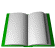SELECTED PUBLICATIONS (with H-index being 49 in ResearcherID or 63 in Google Scholar)

1.       Z Peng, D. Wang and J. Wang, Cooperative dynamic positioning of multiple marine offshore vessels: a modular design, IEEE/ASME Transactions on Mechatronics, vol. 21, in press, 2016.

2.       Y. Shen and J. Wang, Robustness of global exponential stability of nonlinear systems with random disturbances and time delays, IEEE Transactions on Systems, Man and Cybernetics: Systems, vol. 45, in press, 2016.

3.       Q. Liu and J. Wang, L1-minimization algorithms for sparse signal reconstruction based on a projection neural network, IEEE Transactions on Neural Networks and Learning Systems, vol. 27, in press, 2016.

4.       P. Liu, Z. Zeng, and J. Wang, Multistability of recurrent neural networks with non-monotonic activation functions and mixed time delays, IEEE Transactions on Systems, Man and Cybernetics: Systems, vol. 45, in press, 2016.

5.       Y. Xia and J. Wang, A bi-projection neural network for solving constrained quadratic optimization problems, IEEE Transactions on Neural Networks and Learning Systems, vol. 27, no. 2, pp. 214 - 224, 2016.

6.       Q. Liu and J. Wang, A second-order multi-agent network for bound-constrained distributed optimization, IEEE Transactions on Automatic Control, vol. 60, no. 12, pp. 3310-3315, 2015.

7.       B. Tang and J. Wang, and Y Zhang, A delay-distribution approach to stabilization of networked control systems, IEEE Transactions on Control of Network Systems, vol. 2, no. 4, pp. 382 - 392, 2015.

8.       S. Zhang, Y. Xia, and J. Wang, A complex-valued projection neural network for constrained optimization of real functions in complex variables, IEEE Transactions on Neural Networks and Learning Systems, vol. 26, no. 12, pp. 3227 - 3238, 2015.

9.       X. Le and J. Wang, Neurodynamics-based robust pole assignment for high-order descriptor systems, IEEE Transactions on Neural Networks and Learning Systems, vol. 26, no. 11, pp. 2962-2971, 2015.

10.    Q. Liu and J. Wang, A projection neural network for constrained quadratic minimax optimization, IEEE Transactions on Neural Networks and Learning Systems, vol. 26, no. 11, pp. 2891-2900, 2015.

11.    S. Yang, Z. Guo, and J. Wang, Robust synchronization of multiple memristive neural networks with uncertain parameters via nonlinear coupling, IEEE Transactions on Systems, Man and Cybernetics: Systems, vol. 45, no. 7, pp. 1077-1086, 2015.

12.    Y. Xia and J. Wang, Low-dimensional neural network-based Kalman filter for speech enhancement, Neural Networks, vol. 67, pp. 131-139, 2015.

13.    J. Hu and J. Wang, Global exponential periodicity and stability of discrete-time complex-valued recurrent neural networks with time-delays, Neural Networks, vol. 66, pp. 119-130, 2015.

14.    Z. Guo, S. Yang, and J. Wang, Global exponential synchronization of multiple memristive neural networks with time delay via nonlinear coupling, IEEE Transactions on Neural Networks and Learning Systems, vol. 26, no. 6, pp. 1300 - 1311, 2015.

15.    Z. Yan and J. Wang, Nonlinear model predictive control based on collective neurodynamic optimization, IEEE Transactions on Neural Networks and Learning Systems, vol. 26, no. 4, pp. 840-850, 2015.

16.    S. Qin, J. Wang, and X. Xue, Convergence and attractivity of memristor-based cellular neural networks with time delays, Neural Networks, vol. 63, pp. 223-233, 2015.

17.    J. Lian and J. Wang, Passivity of switched recurrent neural networks with time-varying delays, IEEE Transactions on Neural Networks and Learning Systems, vol. 26, no. 2, pp. 257-266, 2015.

18.    Z. Guo, J. Wang, and Z. Yan, Global exponential synchronization of two memristor-based recurrent neural networks with time delays via static or dynamic coupling, IEEE Transactions on Systems, Man and Cybernetics: Systems, vol. 45, no. 2, pp. 235-249, 2015.

19.    G. Li, Z. Yan, and J. Wang, A one-layer recurrent neural network for constrained nonconvex optimization, Neural Networks, vol. 61, pp. 10-21, 2015.

20.    Z. Guo, J. Wang, and Z. Yan, Passivity and passification of memristor-based recurrent neural networks with time-varying delays, IEEE Transactions on Neural Networks and Learning Systems, vol. 25, no. 11, pp. 2099 - 2109, 2014.

21.    J. Fan, J. Wang, and M. Han, Cooperative coevolution for large-scale optimization based on kernel fuzzy clustering and variable trust region methods, IEEE Transactions on Fuzzy Systems, vol. 22, no. 4, pp. 829-839, 2014.

22.    Q. Liu, T. Huang, and J. Wang, One-layer continuous-time and discrete-time projection neural networks for solving variational inequalities and related optimization problems, IEEE Transactions on Neural Networks and Learning Systems, vol. 25, no. 7, pp. 1308-1318, 2014.

23.    Z. Yan, J. Wang, and G. Li, A collective neurodynamic optimization approach to bound-constrained nonconvex optimization, Neural Networks, vol. 55, pp. 20-29, 2014.

24.    Z. Guo, J. Wang, and Z. Yan, A systematic method for analyzing robust stability of interval neural networks with time-delays based on stability criteria, Neural Networks, vol. 54, pp. 112-122, 2014.

25.    Z. Guo, J. Wang, and Z. Yan, Attractivity analysis of memristor-based cellular neural networks with time-varying delays, IEEE Transactions on Neural Networks and Learning Systems, vol. 25, no. 4, pp. 704-717, 2014.

26.    Z. Yan and J. Wang, Robust model predictive control of nonlinear systems with unmodeled dynamics and bounded uncertainties based on neural networks, IEEE Transactions on Neural Networks and Learning Systems, vol. 25, no. 3, pp. 457-469, 2014.

27.    G. Li, Z. Yan, and J. Wang, A one-layer recurrent neural network for constrained nonsmooth invex optimization, Neural Networks, vol. 50, pp. 79-89, 2014.

28.    X. Le and J. Wang, Robust pole assignment for synthesizing feedback control systems using recurrent neural networks, IEEE Transactions on Neural Networks and Learning Systems, vol. 25, no. 2, pp. 383-393, 2014.

29.    Z. Guo, J. Wang, and Z. Yan, Global exponential dissipativity and stabilization of memristor-based recurrent neural networks with time-varying delays, Neural Networks, vol. 48, pp. 158-172, 2013.

30.    A. Hosseini, J. Wang, and S. M. Hosseini, A recurrent neural network for solving a class of generalized convex optimization problems, Neural Networks, vol. 44, pp. 78-86, 2013.

31.    Y. Shen and J. Wang, Robustness analysis of global exponential stability of nonlinear systems with time delays and neutral terms, IET Control Theory and Applications, vol. 7, no. 9, pp. 1227-1232, 2013.

32.    Q. Liu and J. Wang, A one-layer projection neural network for nonsmooth optimization subject to linear equalities and bound constraints, IEEE Transactions on Neural Networks and Learning Systems, vol. 24, no. 5, pp. 812-824, 2013.

33.    Z. Yan and J. Wang, Model predictive control of nonlinear systems with unmodeled dynamics based on feedforward and recurrent neural networks, IEEE Transactions on Industrial Informatics, vol. 8, no. 4, pp. 746-756, 2012.

34.    Z. Yan and J. Wang, Model predictive control for tracking of underactuated vessels based on recurrent neural networks, IEEE Journal of Oceanic Engineering, vol. 37, no. 4, pp. 717-726, 2012.

35.    Y. Pan and J. Wang, Model predictive control of unknown nonlinear dynamical systems based on recurrent neural networks, IEEE Transactions on Industrial Electronics, vol. 59, no. 8, pp. 3089-3101, 2012.

36.    J. Hu and J. Wang, Global stability of complex-valued recurrent neural networks with time-delays, IEEE Transactions on Neural Networks and Learning Systems, vol. 23, no. 6, pp. 853-865, 2012.

37.    D. Li, M. Han, and J. Wang, Chaotic time series prediction based on a novel robust echo state network, IEEE Transactions on Neural Networks and Learning Systems, vol. 23, no. 5, pp. 787-799, 2012.

38.    X. Hu and J. Wang, Solving the assignment problem using continuous-time and discrete-time improved dual networks, IEEE Transactions on Neural Networks and Learning Systems, vol. 23, no. 5, pp. 821-827, 2012.

39.    Q. Liu, Z. Guo, and J. Wang, A one-layer recurrent neural network for constrained pseudoconvex optimization and its application for portfolio optimization, Neural Networks, vol. 26, no. 1, pp. 99-109, 2012.

40.    Y. Shen and J. Wang, Robustness analysis of global exponential stability of recurrent neural networks in the presence of time delays and random disturbances, IEEE Transactions on Neural Networks and Learning Systems, vol. 23, no. 1, pp. 87-96, 2012.

41.    Z. Guo, Q. Liu, and J. Wang, A one-layer recurrent neural network for pseudoconvex optimization subject to linear equality constraints, IEEE Transactions on Neural Networks, vol. 22, no. 12, pp. 1892-1900, 2011.

42.    Q. Liu and J. Wang, A one-layer recurrent neural network for constrained nonsmooth optimization, IEEE Transactions on Systems, Man and Cybernetics - Part B: Cybernetics, vol. 40, no. 5, pp. 1323-1333, 2011.

43.    M. Han, J. Fan, and J. Wang, A dynamic feedforward neural network based on Gaussian particle swarm optimization and its application for predictive control, IEEE Transactions on Neural Networks, vol. 22, no. 9, pp. 1457-1468, 2011.

44.    Q. Liu and J. Wang, Finite-time convergent recurrent neural network with a hard-limiting activation function for constrained optimization with piecewise-linear objective functions, IEEE Transactions on Neural Networks, vol. 22, no. 4, pp. 601-613, 2011.

45.    J. Wang, Analysis and design of k-winners-take-all model with a single state variable and Heaviside step activation function, IEEE Transactions on Neural Networks, vol. 21, no. 9, pp. 1496-1506, 2010.

46.    Z. Zeng and J. Wang, Associative memories based on class of continuous-time cellular neural networks designed using space-invariant cloning templates, Neural Networks, vol. 22, nos.5-6, pp. 651-657, 2009.

47.    T. Mei, F. Yin, and J. Wang, Blind source separation based on cumulants with time and frequency non-propertiesIEEE Transactions on Audio, Speech, and Language Processing, vol. 17, no. 6, pp. 1099-1108, 2009.

48.    Y. Shen and J. Wang, Almost sure exponential stability of recurrent neural networks with Markovian switching, IEEE Transactions on Neural Networks, vol. 20, no. 5, pp. 840-855, 2009.

49.    X. Hu and J. Wang, An improved dual neural network for solving a class of quadratic programming problems and its k-winners-take-all application, IEEE Transactions on Neural Networks, vol. 19, no. 12, pp. 2022-2031, 2008.

50.    Z. Zeng and J. Wang, Analysis and design of high-capacity associative memories based on class of discrete-time recurrent neural networks, IEEE Transactions on Systems, Man and Cybernetics - Part B: Cybernetics, vol. 38, no. 6, pp. 1525-1536, 2008.

51.    W. Lu and J. Wang, Convergence analysis of a class of nonsmooth gradient systems, IEEE Transactions on Circuits and Systems - Part I: Regular Papers, vol. 55, no. 11, pp. 3514-3527, 2008.

52.    Y. Xia, G. Feng, and J. Wang, A novel neural network for solving nonlinear optimization problems with inequality constraints, IEEE Transactions on Neural Networks, vol. 19, no. 8, pp. 1340-1353, 2008.

53.    Q. Liu, and J. Wang, A one-layer recurrent neural network with a discontinuous activation function for linear programming, Neural Computation, vol. 20, no. 5, pp. 1366-1383, 2008.

54.    Q. Liu and J. Wang, A one-layer recurrent neural network with a discontinuous hard-limiting activation function for quadratic programming, IEEE Transactions on Neural Networks, vol. 19, no. 4, pp. 558-570, 2008.

55.    Q. Liu and J. Wang, Two k-winners-take-all networks with discontinuous activation functions, Neural Networks, vol. 21, no. 2-3, pp. 406-413, 2008.

56.    Y. Shen and J. Wang, An improved algebraic criterion for global exponential stability of recurrent neural networks with time-varying delays, IEEE Transactions on Neural Networks, vol. 19, no. 3, pp. 528-531, 2008.

57.    B. Chen and J. Wang, Global exponential periodicity and global exponential stability of a class of recurrent neural networks with various activation functions and time-varying delays, Neural Networks, vol. 20, no. 10, pp. 1067-1080, 2007.

58.    Y. Shen and J. Wang, Noise-induced Stabilization of the recurrent neural networks with mixed time-varying delays and Markovian-switching parameters, IEEE Transactions on Neural Networks, vol. 18, no. 6, pp. 1857-1862, 2007.

59.    X. Hu and J. Wang, Solving generally constrained generalized linear variational inequalities using the general projection neural networks, IEEE Transactions on Neural Networks, vol. 18, no. 6, pp. 1697-1708, 2007.

60.    X. Hu and J. Wang, Design of general projection neural network for solving monotone linear variational inequalities and linear and quadratic optimization problems, IEEE Transactions on Systems, Man and Cybernetics - Part B: Cybernetics, vol. 37, no. 5, pp.1414-1421, 2007.

61.    W. Yu, J. Cao, and J. Wang, An LMI approach to delayed global asymptotic stability of the Cohen-Grossberg neural networks via nonsmooth analysis, Neural Networks, vol. 20, no. 7, pp. 810-818, 2007.

62.    Z. Zeng and J. Wang, Analysis and design of associative memories based on recurrent neural networks with linear saturation activation functions and time-varying delays, Neural Computation, vol. 19, no. 8, pp. 2149-2182, 2007.

63.    X. Hu and J. Wang, A recurrent neural network for solving a class of general variational inequalities, IEEE Transactions on Systems, Man and Cybernetics - Part B: Cybernetics, vol. 37, no. 3, pp. 528-539, 2007.

64.    Z. Zeng and J. Wang, Global exponential stability of recurrent neural networks in the presence of strong external stimuli, Neural Networks, vol. 19, no. 10, pp. 1528-1537, 2006.

65.    S. Liu and J. Wang, A simplified dual neural network for quadratic programming with its KWTA application, IEEE Transactions on Neural Networks, vol. 17, no. 6, pp. 1500-1510, 2006.

66.    X. Hu and J. Wang, Solving pseudomonotone variational inequalities and pseudoconvex optimization problems using the projection neural network, IEEE Transactions on Neural Networks, vol. 17, no. 6, pp. 1487-1499, 2006.

67.    Z. Zeng and J. Wang, Multiperiodicity of discrete-time delayed neural networks evoked by periodic external inputs, IEEE Transactions on Neural Networks, vol. 17, no. 5, pp. 1141-1151, 2006.

68.    Z. Zeng and J. Wang, Improved conditions for global exponential stability of recurrent neural networks with time-varying delays, IEEE Transactions on Neural Networks, vol. 17, no. 3, pp. 623-635, 2006.

69.    Z. Zeng and J. Wang, Complete stability of cellular neural networks with time-varying delays, IEEE Transactions on Circuits and Systems - Part I: Regular Papers, vol. 53, no. 4, pp. 944-955, 2006.

70.    Z. Zeng and J. Wang, Multiperiodicity and exponential attractivity evoked by periodic external inputs in delayed cellular neural networks, Neural Computation, vol. 18, no. 4, pp. 848-870, 2006.

71.    S. Hu and J. Wang, Global robust stability of a class of discrete-time interval neural networks, IEEE Transactions on Circuits and Systems - Part I: Regular Papers, vol. 53, no. 1, pp. 129- 138, 2006.

72.    Y. Li and J. Wang, A network model for blind source extraction in various ill-conditioned cases, Neural Networks, vol. 18, no. 10, pp. 1348-1356, 2005.

73.     B. Chen and J. Wang, Global exponential periodicity of a class of recurrent neural networks with oscillating parameters and time-varying delays, IEEE Transactions on Neural Networks, vol. 16, no. 6, pp. 1440-1448, 2005.

74.    X. Liao, J. Wang, and Z. Zeng, Global asymptotic stability and global exponential stability of delayed cellular neural networks, IEEE Transactions on Circuits and Systems - Part II: Express Briefs, vol. 52, no. 7, pp. 403-409, 2005.

75.    J. Cao and J. Wang, Global exponential stability and periodicity of recurrent neural networks with time delays, IEEE Transactions on Circuits and Systems - Part I: Regular Papers, vol. 52, no. 5, pp. 920-931, 2005.

76.    Z. Zeng, J. Wang, and X. Liao, Global exponential stability of a general class of recurrent neural networks with unbounded time-varying delays, IEEE Transactions on Circuits and Systems - Part II: Express Briefs, vol. 52, no. 3, pp. 168-173, 2005.

77.    Y. Xia and J. Wang, Recurrent neural networks for solving nonlinear convex programs with linear constraints, IEEE Transactions on Neural Networks, vol. 16, no 2, pp. 379-386, 2005.

78.    J. Cao and J. Wang, Global asymptotic and robust stability of recurrent neural networks with time delays, IEEE Transactions on Circuits and Systems - Part I: Regular Papers, vol. 52, no. 2, pp. 417-426, 2005.

79.    Y. Xia, G. Feng, and J. Wang, A primal-dual  neural  network for on-line resolving constrained  kinematic redundancy in robot motion control, IEEE Transactions on Systems, Man and Cybernetics - Part B: Cybernetics, vol. 35, no. 1, pp. 54-64, 2005.

80.    Z. Zeng, J. Wang, and X. Liao, Stability analysis of delayed cellular neural networks described using cloning templates, IEEE Transactions on Circuits and Systems - Part I: Regular Papers, vol. 51, no. 11, pp. 2313-2324, 2004.

81.    Y. Xia, G. Feng, and J. Wang,  A recurrent neural network with exponential convergence for solving convex quadratic program and linear piecewise equations, Neural Networks, vol. 17, no. 7, pp. 1003-1015, 2004.

82.    Y. Li, J. Wang, and A. Cichocki, Blind source extraction from convolutive mixtures in ill-conditioned multi-input multi-output channels, IEEE Transactions on Circuits and Systems - Part I: Regular Papers, vol. 51, no. 9, pp. 1814-1822, 2004.

83.    Y. Xia and J. Wang, A recurrent neural network for nonlinear convex optimization subject to nonlinear inequality constraints, IEEE Transactions on Circuits and Systems - Part I: Regular Papers, vol. 51, no. 7, pp. 1385-1394, 2004.

84.    Y. Xia, J. Wang, and L.-M. Fok, Grasping force optimization of multi-fingered robotic hands using a recurrent neural network, IEEE Transactions on Robotics and Automation, vol. 20, no. 3, pp. 549-554, 2004.

85.    L. Tian, J. Wang, and Z. Mao, Constrained motion control of flexible robot manipulators based on recurrent neural networks, IEEE Transactions on Systems, Man and Cybernetics - Part B: Cybernetics, vol. 34, no. 3, pp. 1541-1552, 2004.

86.    D. Liu, S. Hu, and J. Wang, Global output convergence of a class of continuous-time recurrent neural networks with time-varying thresholds, IEEE Transactions on Circuits and Systems - Part II: Express Briefs, vol. 51, pp. 161-167, 2004.

87.    Y. Xia and J. Wang, A one-layer recurrent neural network for support vector machine learning, IEEE Transactions on Systems, Man and Cybernetics - Part B: Cybernetics, vol. 34, no. 2, pp. 1261-1269, 2004.

88.    J. Cao and J. Wang, Absolute exponential stability of recurrent neural networks with time delays and Lipschitz-continuous activation functions, Neural Networks, vol. 17, no. 3, pp. 379-390, 2004.

89.    Y. Tan and J. Wang, A support vector machine with a hybrid kernel and minimal Vapnik-Chervonenkis dimension, IEEE Transactions on Knowledge and Data Engineering, vol. 16, no. 4, pp. 385-395, 2004.

90.    Y. Xia and J. Wang, A general projection neural network for solving optimization and related problemsIEEE Transactions on Neural Networks, vol. 15, pp. 318-328, 2004.

91.    Y. Zhang and J. Wang, Obstacle avoidance for kinematically redundant manipulators using a dual neural network, IEEE Transactions on Systems, Man and Cybernetics - Part B: Cybernetics, vol. 34, no. 1, pp. 752-759, 2004.

92.    X. Zhu, H. Ding, and J. Wang, Grasp analysis and synthesis based on a new quantitative measure, IEEE Transactions on Robotics and Automation, vol. 19, no. 6, pp. 942-953, 2003.

93.    S. Hu and J. Wang, Multilayer recurrent neural networks for on-line robust pole assignment, IEEE Transactions on Circuits and Systems - Part I: Fundamental Theory and Applications, vol. 50, no. 11, pp. 1488-1496, 2003.

94.    Z. Zeng, J. Wang, and X. Liao, Global exponential stability of a general class of recurrent neural networks with time-varying delays, IEEE Transactions on Circuits and Systems - Part I: Fundamental Theory and Applications, vol. 50, no. 10, pp. 1353-1358, 2003.

95.    J. Wang, Formation of machine cells and part families in cellular manufacturing systems using a linear assignment algorithm, Automatica, vol. 39, no. 9, pp. 1607-1615,  2003.

96.    Y. Zhu and J. Wang, Synthesis of force-closure grasps on 3-D objects based on the Q-distance, IEEE Transactions on Robotics and Automation, vol. 19, no. 4, pp. 669-679, 2003.

97.    X. Liao and J. Wang, Global dissipativity of continuous-time recurrent neural networks with time delayPhysical Review E, vol. 68, no. 1, pp. 016118.1-7, 2003.

98.    Y. Zhang, J. Wang, and Y. Xia, A dual neural network for redundancy resolution of kinematically redundant manipulators subject to joint limits and joint velocity limits, IEEE Transactions on Neural Networks, vol. 14, no. 3, pp. 658-667, 2003.

99.    X. Liao and J. Wang, Algebraic criteria for global exponential stability of cellular neural networks with multiple time delays, IEEE Transactions on Circuits and Systems - Part I: Fundamental Theory and Applications, vol. 50, no. 2, pp. 268-275, 2003.

100.J. Cao and J. Wang, Global asymptotic stability of a general class of recurrent neural networks with time-varying delaysIEEE Transactions on Circuits and Systems - Part I: Fundamental Theory and Applications, vol. 50, no. 1, pp. 34-44, 2003.

101.S. Hu and J. Wang, Absolute exponential stability of a class of continuous-time recurrent neural networks, IEEE Transactions on Neural Networks, vol. 14, no. 1, pp. 35-45, 2003.

102.Y. Zhang, J. Wang, and Y. Xu, A dual neural network for bi-criteria kinematic control redundant manipulators, IEEE Transactions on Robotics and Automation, vol. 18, no. 6, pp. 923-931, 2002.

103. Z. He, Y. Zhang, C. Wei, and J. Wang, A multi-stage self-organizing algorithm combined transiently chaotic neural network for cellular channel assignment, IEEE Transactions on Vehicular Technology, vol. 51, no. 6, pp. 1386-1396, 2002.

104. S. Hu and J. Wang, A gradient flow approach to on-line robust pole assignment for synthesizing output feedback control systems, Automatica, vol.. 38, no. 11, pp. 1959-1968, 2002.

105. Y. Zhang and J. Wang, A dual neural network for constrained torque optimization of kinematically redundant manipulators, IEEE Transactions on Systems, Man and Cybernetics - Part B: Cybernetics, vol. 32, no. 5, pp. 654-662, 2002.

106. Y. Zhang, D. Jiang, and J. Wang, A recurrent neural network for solving Sylvester equation with time-varying coefficients, IEEE Transactions on Neural Networks, vol. 13, no. 5, pp. 1053-1063, 2002.

107. S. Hu and J. Wang, Global stability of a class of continuous-time recurrent neural networks, IEEE Transactions on Circuits and Systems - Part I: Fundamental Theory and Applications, vol. 49, no. 9, pp. 1334-1347, 2002.

108. S. Hu and J. Wang, Global stability of a class of discrete-time recurrent neural networks, IEEE Transactions on Circuits and Systems - Part I: Fundamental Theory and Applications, vol. 49, no. 8, pp. 1104-1117, 2002.

109. M. Xiong, J. Wang, and P. Wang, Differential-algebraic approach to linear programming, Journal of Optimization Theory and Applications, vol. 114, no. 2, pp. 443-470, 2002.

110. Y. Li and J. Wang,  Sequential blind extraction of instantaneously mixed sources, IEEE Transactions on Signal Processing, vol. 50, no. 5,  pp. 997-1006, 2002.

111. S. Hu and J. Wang, Global asymptotic stability and global exponential stability of continuous-time recurrent neural networks, IEEE Transactions on Automatic Control, vol. 46, no. 5, pp. 802-807, 2002.

112. Y. Zhang and J. Wang,  Global exponential stability of recurrent neural networks for synthesizing linear feedback control systems via pole assignment, IEEE Transactions on Neural Networks, vol. 13, no. 3, pp. 633-644, 2002.

113. Y. Xia, H. Leung, and J. Wang, A projection neural network and its application to contained optimization problems, IEEE Transactions on Circuits and Systems - Part I: Fundamental Theory and Applications, vol. 49, no. 4, pp. 447-458, 2002.

114. S. Hu and J. Wang, Global exponential stability of continuous-time interval neural networks, Physical Review E, vol. 65, no. 3, pp.036133.1-036133.9, 2002.

115. D. Jiang and J. Wang, Augmented gradient flows for on-line robust pole assignment via state and output feedback, Automatica, vol. 38, no. 2, pp. 279-286, 2002.

116. Y. Tan and J. Wang, Nonlinear blind source separation using higher-order statistics and a genetic algorithm, IEEE Transactions on Evolutionary Computation, vol. 5, no. 6, pp. 600-612, 2001.

117. X. Liang and J. Wang, An additive diagonal stability condition for absolute exponential stability of a general class of neural networks, IEEE Transactions on Circuits and Systems - Part I: Fundamental Theory and Applications, vol. 48, no. 11, pp. 1308-1317, 2001.

118. Y. Zhang and J. Wang, Recurrent neural networks for nonlinear output regulation, Automatica, vol. 37, no. 8, pp. 1161-1173, 2001.

119. Y. Xia and J. Wang, Global asymptotic and exponential stability of a dynamic neural system with asymmetric connection weights, IEEE Transactions on Automatic Control, vol. 46, no. 4, pp. 635-638, 2001.

120.

121. Y. Xia and J. Wang, A dual neural network for kinematic control of redundant robot manipulators, IEEE Transactions on Systems, Man and Cybernetics - Part B: Cybernetics, vol. 31, no. 1, pp. 147-154, 2001.

122. Y. Tan, J. Wang, and J. M. Zurada, Nonlinear blind source separation using a radial basis function network, IEEE Transactions on Neural Networks, vol.  12, no. 1, pp. 124-134, 2001.

123. S. Hu and J. Wang, Quadratic stabilizability of a new class of linear systems with structural independent time-varying uncertainties, Automatica, vol. 37, no. 1, pp. 51-59, 2001

124. S. Hu and J. Wang, On stabilization of a new class of linear time-invariant interval systems via constant state feedback control, IEEE Transactions on Automatic Control, vol. 45, no. 11, pp. 2106-2111, 2000.

125. Y. Xia and J. Wang, A discrete-time recurrent neural network for shortest-path routing, IEEE Transactions on Automatic Control, vol. 45, no. 11, pp.2129-2135, 2000.

126. X. Liang and J. Wang, A recurrent neural network for nonlinear optimization with a continuously differentiable objective function and bound constraints, IEEE Transactions on Neural Networks, vol. 11, no. 6, pp. 1251-1262, 2000.

127. D. Jiang and J. Wang,  On-line learning of dynamic systems in the presence of model mismatch and disturbance, IEEE Transactions on Neural Networks, vol. 11, no., 6, pp. 1272-1283, 2000.

128. Y. Li, J. Wang, and J. M. Zurada, Blind extraction of singularly mixed source signals, IEEE Transactions on Neural Networks, vol.  11, no., 6, pp. 1413-1422, 2000.

129. X. Li, S. K. Tso, and J. Wang, Real-time tool condition monitoring using wavelet transform and fuzzy techniques, IEEE Transactions on Systems, Man and Cybernetics - Part C: Applications and Reviews, vol. 31, no. 3, pp. 352-357, 2000.

130. X. Liang and J. Wang, Absolute exponential stability of neural networks with a general class of activation functions, IEEE Transactions on Circuits and Systems - Part I: Fundamental Theory and Applications, vol. 47, no. 8, pp. 1258-1263, 2000.

131. Y. Xia and J. Wang, Global exponential stability of recurrent neural networks for solving optimization and related problems, IEEE Transactions on Neural Networks, vol. 11, no. 4,  pp. 1017-1022,  2000.

132. Y. Xia and J. Wang, On the stability of globally projected dynamical systems, Journal of Optimization Theory and Applications, vol. 106, no. 1, pp. 129-150, 2000.

133. D. Jiang and J. Wang,  A recurrent neural network for on-line design of robust optimal filters, IEEE Transactions on Circuits and Systems - Part I: Fundamental Theory and Applications, vol. 47, no. 6, pp. 921-926,  2000.

134. Y. Xia and J. Wang, A recurrent neural network for solving linear projection equations, Neural Networks, vol. 13, no. 3, pp.337-350, 2000.

135. X. Liang and J. Wang, A proof of Kaszkurwicz and Bhara's conjecture on absolute stability of neural networks in two-neuron case, IEEE Transactions on Circuits and Systems - Part I: Fundamental Theory and Applications, vol. 47, no. 4, pp. 609-611, 2000.

136. W. S. Tang and J. Wang, Two recurrent neural networks for joint torque optimization of kinematically redundant manipulators, IEEE Transactions on Systems, Man and Cybernetics - Part B: Cybernetics, vol. 30, no. 1, pp. 120-128, 2000.

137. J. Wang, Q. Hu, and D. Jiang, A  Lagrangian network for kinematic control of redundant robot manipulators, IEEE Transactions on Neural Networks, vol. 10, no. 5, pp. 1123-1132, 1999.

138. H. Ding and J. Wang, Recurrent neural networks for minimum infinity-norm kinematic control of redundant manipulators, IEEE Transactions on Systems, Man, and Cybernetics - Part A: Systems and Humans, vol. 29, no. 3, pp. 269-276, 1999.

139. Y. Xia, J. Wang, and D. L. Hung, Recurrent neural networks for solving linear inequalities and equations, IEEE Transactions on Circuits and Systems - Part I: Fundamental Theory and Applications, vol. 46, no. 4, pp. 452-462, 1999.

140. J. Wang, A linear assignment clustering algorithm based on the least similar cluster representatives, IEEE Transactions on Systems, Man, and Cybernetics - Part A: Systems and Humans, vol. 29, no. 1, pp. 100-104, 1999.

141. D. Jiang and J. Wang, A recurrent neural network for real-time semi-definite programming, IEEE Transactions on Neural Networks, vol. 10, no. 1, pp. 81-93, 1999.

142. Y. Xia and J. Wang, A general methodology for designing globally convergent optimization neural networks, IEEE Transactions on Neural Networks, vol. 9, no. 6, pp. 1331-1343, 1998.

143. J. Wang, Primal and dual neural networks for shortest-path routing, IEEE Transactions on Systems, Man, and Cybernetics - Part A: Systems and Humans, vol. 28, no. 6, pp. 864-869, 1998.

144. J. Wang and G. Wu, A multilayer recurrent neural network for solving continuous-time algebraic Riccati equations, Neural Networks, vol. 11, no. 5, pp. 939-950, 1998.

145. J. Wang and Y. Xia, Analysis and design of primal-dual assignment networks, IEEE Transactions on Neural Networks, vol. 9, no. 1, pp. 183-194, 1998.

146. J. Wang, Recurrent neural networks for computing pseudoinverses of rank-deficient matrices, SIAM Journal on Scientific Computing, vol.18, no. 5, pp. 1479-1493, 1997.

147. J. Wang, Primal and dual assignment networks, IEEE Transactions on Neural Networks, vol. 8, no. 3, pp. 784-790, 1997.

148. J. Wang and C. Roze, Formation of machine cells and part families: a modified p-median formulation and a comparative study, International Journal of Production Research, vol. 35, no. 5, pp. 1269-1286, 1997.

149. J. Wang, A recurrent neural network for solving the shortest path problem, IEEE Transactions on Circuits and Systems - Part I: Fundamental Theory and Applications, vol. 43, no. 6, pp. 482-486, 1996.

150. J. Wang and G. Wu, A multilayer recurrent neural network for on-line synthesis of minimum-norm linear feedback control systems via pole assignment, Automatica, vol. 32, no. 3, pp. 435-442, 1996.

151. J. Wang, Analysis and design of an analog sorting network, IEEE Transactions on Neural Networks, vol. 5, no. 4, pp. 962-971, 1995.

152. J. Wang, A deterministic annealing neural network for convex programming, Neural Networks, vol. 7, no. 4, pp. 629-641, 1994.

153. J. Wang, Artificial neural networks versus natural neural networks: a connectionist paradigm for preference assessment, Decision Support Systems,  vol. 11, no. 5, pp. 415-429, 1994.

154. J. Wang, Analysis and design of a recurrent neural network for linear programming, IEEE Transactions on Circuits and Systems - Part I: Fundamental Theory and Applications, vol. 40, no. 9, pp. 613-618, 1993.##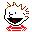INVITED LECTURES

• Numerous keynote/plenary/invited talks at numerous conferences/symposia/workshops in Asia (Brunei, China, India, Indonesia, Japan, Korea, Kuwait, Malaysia, Maldives, Nepal, Philippines, Qatar, Singapore, Thailand, Turkey, United Arab Emirates, Vietnam), Europe (Bulgaria, Czech, France, Germany, Hungary, Lithuania, Malta, Poland, Russia, Serbia, Slovakia, Spain, UK), North America (USA, Mexico), Africa (Egypt), and Oceania (Australia and New Zealand).
• IEEE Computational Intelligence Society Distinguished Lectures at several universities in Australia, France, India, Italy, Serbia, Singapore, Spain, and Tunisia.
• Seminars at numerous universities, research institutes and laboratories all over the world.